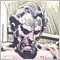# Efficiently assign an Array434

If I have 2+ arrays and I want to edit etc one of the arrays, how do I efficiently assign the array so I can make changes?

Lets say I have two arrays and I want to change j to 10, and I've used some process to chose array "j" ....

```int i[]={1,2,3};
int j[]={1,2};
string arraytouse="j";```

I don't want to use an if statement, as I would need to repeat the code for each array? And I can't assign a variable to the array.

```if(arraytouse=="i")
i=10;
if(arraytouse=="j")
j=10;

// This wont work!
arraytouse=10;
```

This does work ok; ArrayCopy of the selected array, make changes to the copy, then ArrayCopy back to the original .

```int copy[];
if(arraytouse=="i")ArrayCopy(copy,i);
if(arraytouse=="j")ArrayCopy(copy,j);

copy=10;  // use once for whatever array assigned

if(arraytouse=="i")ArrayCopy(i,copy);
if(arraytouse=="j")ArrayCopy(j,copy);
```

But that seems a bit cumbersome, for probably a simple task. Is there a better way?3304

```#property strict

int i[]={1,2,3};
int j[]={1,2};

typedef void (*TVoidFunc)(const int index, const int value);

TVoidFunc        ptrArraySetter = NULL;            // ptr to the array setter

void OnStart()
{
// and I've used some process to chose array "j"
ptrArraySetter = ChangeJ;

// Change it
ptrArraySetter(0,10);

// Prove it
PrintFormat("j should be 10. It is [%d]", j);
}

void ChangeI(const int index, const int value)
{
i[index] = value;
}

void ChangeJ(const int index, const int value)
{
j[index] = value;
}
```1647

Anthony Garot:

I think it's very debatable whether that's less cumbersome than the original code. Still requires a set of if conditions to assign the correct value to ptrArraySetter, and adds a requirement for each array to have a setter function. If there needed to be a getter as well as a setter, plus the multiple arrays which andrew mentions, then things start multiplying unpleasantly.

In the absence of a Javascript-like langauge where we could do...

```var arrays = {
i: [1, 2, 3],
j: [1, 2]
};
...
var array_to_use = "i";
arrays[array_to_use] = 10;```

... then the best answer probably depends on exactly how andrew needs to read and write the data.

It may simply be a case for a multi-dimensional array:

```int arrays;
// Assignment of initial data into the arrays gets ugly, but access is then potentially easier.
// The current "i" becomes [x] and the current "j" becomes [x]
int array_to_change = 0;
arrays[array_to_change] = 10;

```

Or there may be a case for wrapping the array/data in a class, and making changes via object pointers.11680

JC:

I think it's very debatable whether that's less cumbersome than the original code. Still requires a set of if conditions to assign the correct value to ptrArraySetter, and adds a requirement for each array to have a setter function. If there needed to be a getter as well as a setter, plus the multiple arrays which andrew mentions, then things start multiplying unpleasantly.

In the absence of a Javascript-like langauge where we could do...

... then the best answer probably depends on exactly how andrew needs to read and write the data.

It may simply be a case for a multi-dimensional array:

Or there may be a case for wrapping the array/data in a class, and making changes via object pointers.

```'j' - pointer to this function type is not supported yet        ArrayFill.mq5   36      62
```
I tried ...38725

Anthony Garot:
JC:
...

Why all these complications ? I missed something maybe.

```void OnStart()
{
int i[]= {1,2,3};
int j[]= {1,2};

SetArrayValue(i,0,10);
SetArrayValue(j,0,10);
}
void SetArrayValue(int array[],int index,int value)
{
if(index>=ArraySize(array)) return;

array[index]=value;
}```3304

Alain Verleyen:

Why all these complications ?

OP seemed to want a solution using a pointer to an array, so I showed a way that could be done.

As you have shown, and JC intimated, there are other ways to tackle the problem. The OP now has many options.

:-D434

Thanks all. As Anthony says, I think, there are many different solution depending on the problems. I liked Alain's as I could understand it straight away!